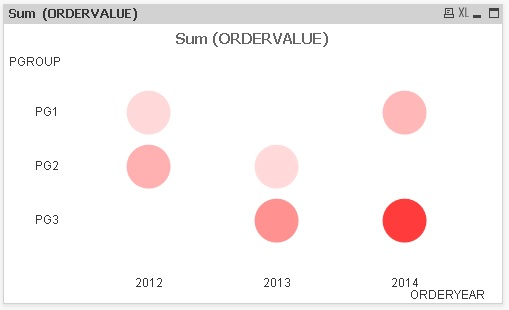QlikView App Dev

Discussion Board for collaboration related to QlikView App Development.

Announcements
QlikWorld 2022, LIVE in Denver CO., May 16-19, 2022. REGISTER NOW TO RECEIVE EARLY BIRD PRICING
cancel
Showing results for
Did you mean:Contributor III

Coloured Grid Chart modifying Luminosity parameter in HSL

Hello,

I want to build a Grid Chart showing which Product groups were sold, over a range of 3 years.

Please consider the example in the attachment. What I need is a chart like the following:BUT the colors of circles will be set using the function HSL (hue, saturation, luminosity) keeping fixed hue and saturation parameters, and modifying the Luminosity parameter. this will result in a "light" circle for small values, "dark" circles for higher values.

As the luminosity should be set up using a linear function, I found the needed one:

y=(170*x+600-230*MAX)/(10-MAX) where x represent the Sum(OrderValue) and MAX the biggest element in the grid chart.

x is the sum(OrderValue), MAX is the maximum value between all sum(OrderValue)  and y is the luminosity to be calculated,.

So, for Sum(ORDERVALUE)=10 we will have a luminosity=230, for the MAX we will have a luminosity=60 (darkest).

My problem is how to calculate the MAX parameter in this equation (because of my poor knowledge of aggregation functions!).

How to setup the calculus?

Many Thanks!

I understand in a grid chart using a "scale color" on the circles is the only way to give an idea about the circle values. In fact, a grid chart with variable-sized circles is not convenient when values differ too much. Little values generate invisible circles in this case; I do not know other ways to change size of circles in a grid chart i.e using a formula. Is that right? Thanks for any indications

1 Solution

Accepted SolutionsMVP

MAX would be defined as:

max(TOTAL aggr(Sum(ORDERVALUE),ORDERYEAR,PGROUP))

Thanks for including a sample qvw. Makes it much easier to answer your question.

-Rob

http://robwunderlich.com

3 RepliesMVP

MAX would be defined as:

max(TOTAL aggr(Sum(ORDERVALUE),ORDERYEAR,PGROUP))

Thanks for including a sample qvw. Makes it much easier to answer your question.

-Rob

http://robwunderlich.comContributor III
Author

Thank you Rob for you precious help! I have to study better aggr()...!Here the qvd resulting, with two variations and a modification respect to my original thinking: I used rgb() instead of hsl()MVP

Looks nice, Another approach I use is agrb() with a single color and just vary the "A" value.

-RobCommunity Browser